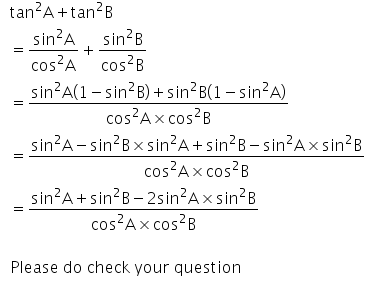# Tan^2A+tan^2B=(sin^2A-sin^2B)/cos^2A-cos^2B

Tan2A+tan2B=(sin2A-sin2B)/cos2A-cos2B